# 高效用项集挖掘(HUI-Miner)

## 样本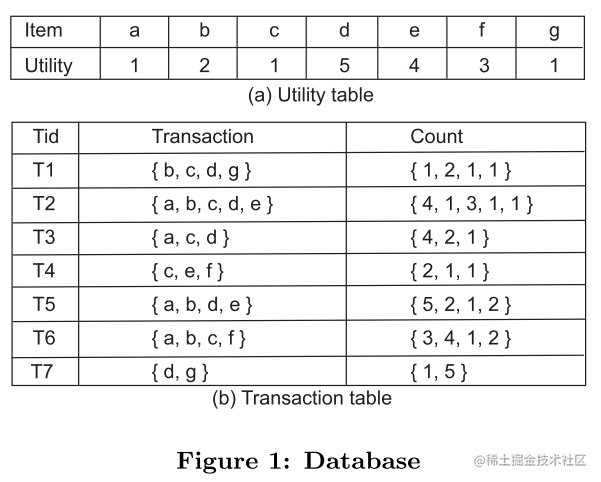$I = {\{i_1, i_2, \dots, i_n\}}$ 每个$i$在效用表($utility \; table$)中都存在对应的效用值($utility \; value$)

$X$是关于$i$的集合，叫效用集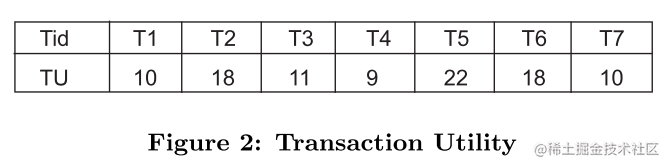$tid$：交易表中的每一项的$id$，且每项都是由$i$组成的超集，交易效用值是所有$i$的效用值的合计

$eu(i)$$i$的效用值

$iu(i, T)$$i$在交易表$T_x$项中出现的次数

$u(i, T)$$u(i, T) = iu(i, T) \times eu(i)$，交易项集$T_x$项中所有$i$的总效用值和

$u(X, T)$$u(X, T) = \sum_{i \in X \land X \subseteq T}u(i, T)$，效用集$X$的所有元素$i$在交易表$T$中的总效用值

$u(X)$$u(X) = \sum_{T \in DB \land X \subseteq T}u(X, T)$，效用项集$X$在数据库$DB$中的总效用值

$tu(T)$$tu(T) = \sum_{i \in T}u(i, T)$，在数据库$DB$中所有$i$$T_x$项的效用值和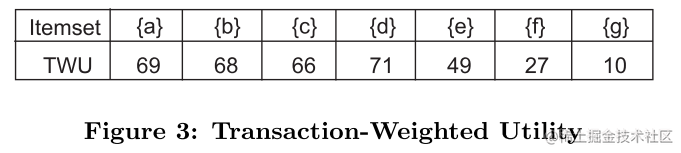$twu(X)$$twu(X) = \sum_{T \in DB \land X \subseteq T}tu(T)$，数据库DB中包含集合X出现在效用集$T_x$项的所有交易项集的总效用值

Ps.其实个人认为加上这个是整个算法的前提，因为它解决了之前项集效用值既不是单调也不是反单调的问题，使整个项集又有了排序的可能，再者产生剩余效用项集，构造剩余效用列表(本文亮点)

### 构造效用列表

#### 初始化效用列表(一元)

$T/X$$X \subseteq T$，在T中项集X之后的所有项都用T/X表示(因为之前的已经排好序了)，例如$T2 / \{eb\} = \{ad\}, T2 / \{c\} = \{bad\}$，参考上表(枚举树会更容易理解)

$ru(X, T)$：是在各交易项中除去共同前缀项集剩下的项的效用值之和，计算公式为：$ru(X, T) = \sum_{i \in (T/X)}u(i, T)$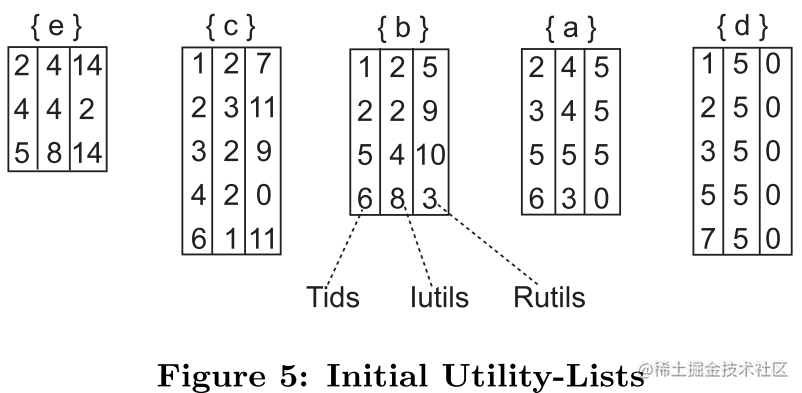$Tids$：包含集合X的交易项id

$Iutils$$u(X, T)$

$Rutils$$ru(X, T)$

#### 二元效用表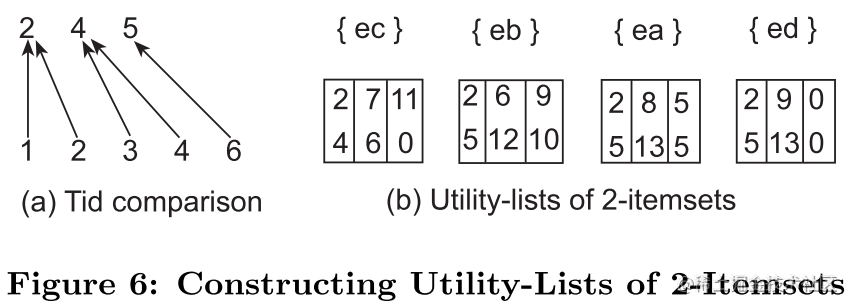• 每一项的Iutils取两者Iutils的和，Rutils取两者中小的Rutils(对照Fig.5，因为它们都是排好序的，前面的项与后面的项结合，必然是会导致剩余项的效用值减少(Rutils)，剩下没合并的项少了)
• Iutils这一列的值相加得到的和就是该项集的效用值
• iutils和rutils这两列的值相加得到的就是该项集的扩展项集的ru值

#### 多元效用表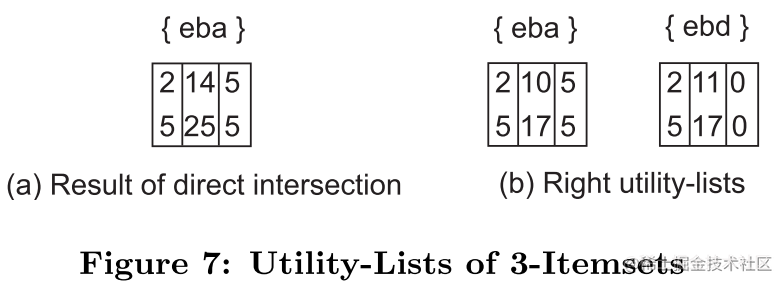## HUI-Miner算法

### 检索空间

1. 根节点为空集
2. 第一层节点为一元项集
3. 从第二层开始，在每个节点末尾依次添加twu值大于父节点最后一位元素twu值的元素。(可以简单地认为把后面的节点单个地添加到前面去，因为每层节点twu值都是有序的)
4. 重复第三步，直到生成所有节点(每层节点不出现重复节点)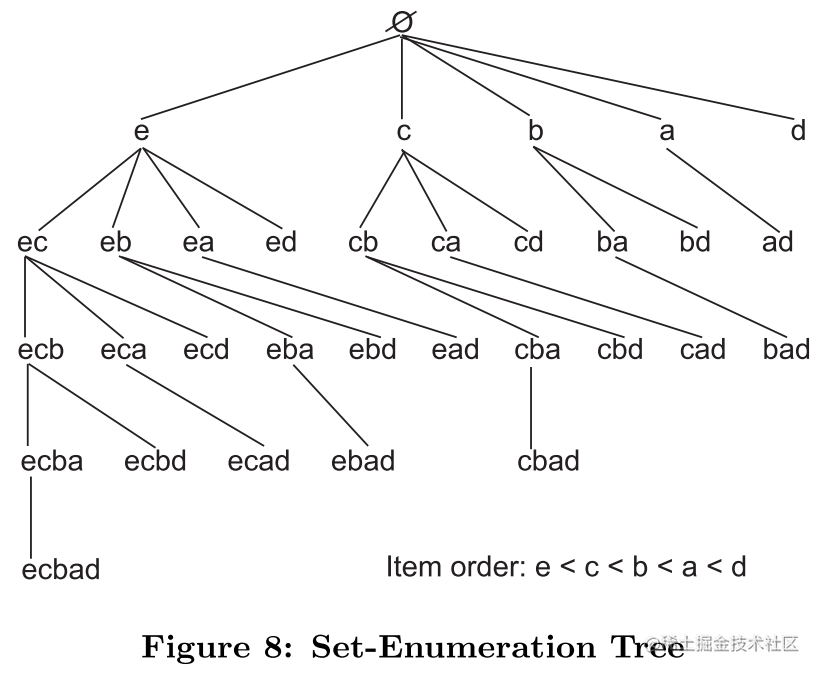### 剪枝策略

1. 若该项目集对应效用列表所有iutils的总和大于阀值，则该项集为高效用项集。
2. 若该项目集对应效用列表所有iutils与rutils的总和大于阀值，则该项目集需要进一步判定。
3. 若该项目集对应效用列表所有iutils与rutils的总和小于阀值，则该项目集及其所有扩展都为低效用，对其进行剪枝。

### 算法实现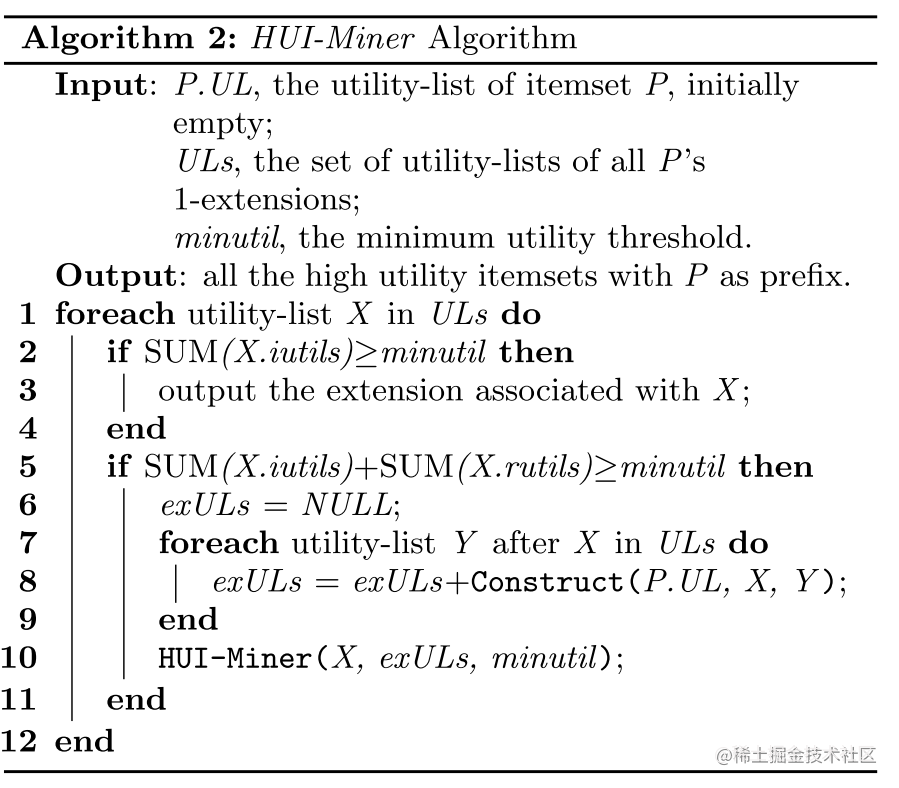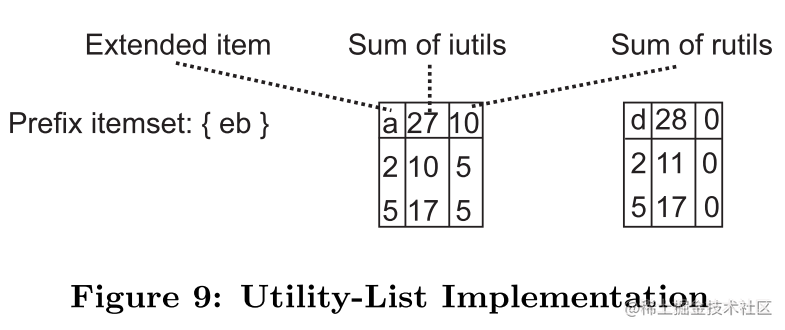# 参考：

1.Mengchi Liu, Junfeng Qu：Mining High Utility Itemsets without Candidate Generation